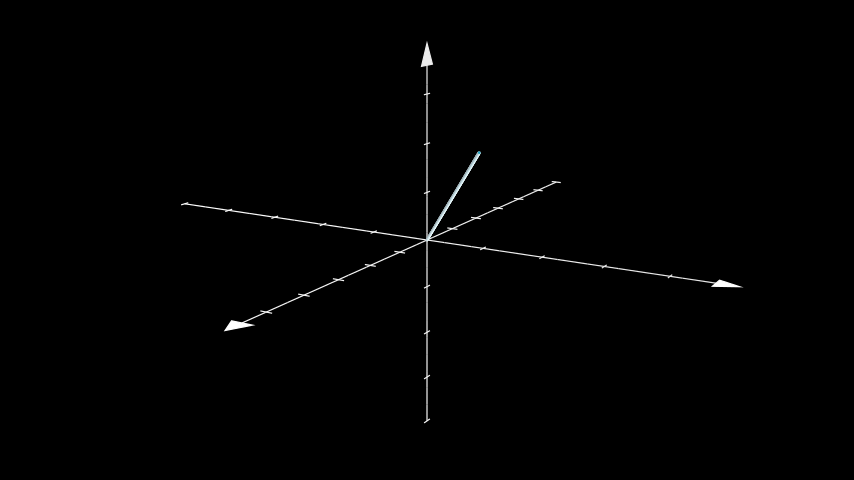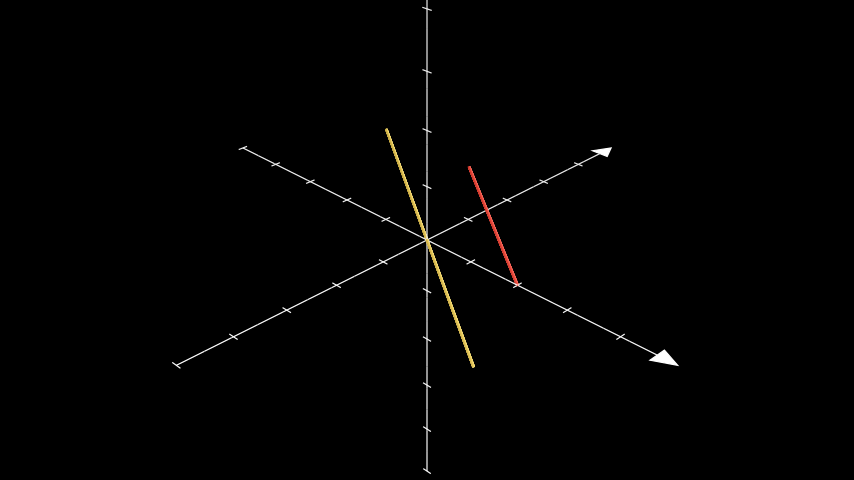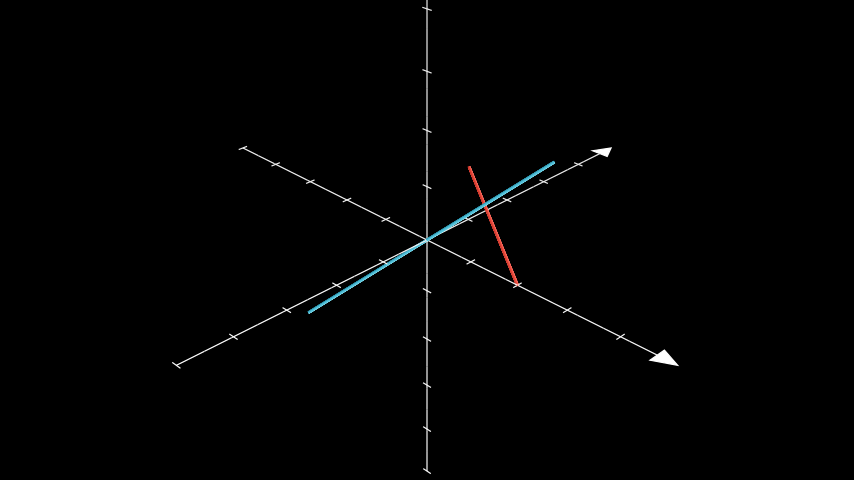# Line3D#

Qualified name: `manim.mobject.three\_d.three\_dimensions.Line3D`

class Line3D(start=array([- 1., 0., 0.]), end=array([1., 0., 0.]), thickness=0.02, color=None, **kwargs)[source]#

Bases: `Cylinder`

A cylindrical line, for use in ThreeDScene.

Examples

Example: ExampleLine3D```from manim import *

class ExampleLine3D(ThreeDScene):
def construct(self):
axes = ThreeDAxes()
line = Line3D(start=np.array([0, 0, 0]), end=np.array([2, 2, 2]))
self.set_camera_orientation(phi=75 * DEGREES, theta=30 * DEGREES)
```
Parameters
• start (`numpy.array`) – The start position of the line.

• end (`numpy.array`) – The end position of the line.

• thickness (`float`) – The thickness of the line.

Methods

 `get_end` Returns the point, where the stroke that surrounds the `Mobject` ends. `get_start` Returns the point, where the stroke that surrounds the `Mobject` starts. `parallel_to` Returns a line parallel to another line going through a given point. `perpendicular_to` Returns a line perpendicular to another line going through a given point. `pointify` `set_start_and_end_attrs` Sets the start and end points of the line.

Attributes

 `animate` Used to animate the application of any method of `self`. `animation_overrides` `color` `depth` The depth of the mobject. `fill_color` If there are multiple colors (for gradient) this returns the first one `height` The height of the mobject. `sheen_factor` `stroke_color` `width` The width of the mobject.
get_end()[source]#

Returns the point, where the stroke that surrounds the `Mobject` ends.

get_start()[source]#

Returns the point, where the stroke that surrounds the `Mobject` starts.

classmethod parallel_to(line, point=array([0., 0., 0.]), length=5, **kwargs)[source]#

Returns a line parallel to another line going through a given point.

Parameters
• line (Line3D) – The line to be parallel to.

• point (Sequence[float]) – The point to pass through.

• kwargs – Additional parameters to be passed to the class.

• length (float) –

Examples

Example: ParallelLineExample```from manim import *

class ParallelLineExample(ThreeDScene):
def construct(self):
self.set_camera_orientation(PI / 3, -PI / 4)
ax = ThreeDAxes((-5, 5), (-5, 5), (-5, 5), 10, 10, 10)
line1 = Line3D(RIGHT * 2, UP + OUT, color=RED)
line2 = Line3D.parallel_to(line1, color=YELLOW)
```
classmethod perpendicular_to(line, point=array([0., 0., 0.]), length=5, **kwargs)[source]#

Returns a line perpendicular to another line going through a given point.

Parameters
• line (Line3D) – The line to be perpendicular to.

• point (Sequence[float]) – The point to pass through.

• kwargs – Additional parameters to be passed to the class.

• length (float) –

Examples

Example: PerpLineExample```from manim import *

class PerpLineExample(ThreeDScene):
def construct(self):
self.set_camera_orientation(PI / 3, -PI / 4)
ax = ThreeDAxes((-5, 5), (-5, 5), (-5, 5), 10, 10, 10)
line1 = Line3D(RIGHT * 2, UP + OUT, color=RED)
line2 = Line3D.perpendicular_to(line1, color=BLUE)
If either `start` or `end` are `Mobjects`, this gives their centers.